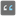• # Int Object is Not Iterable

## Question related to missionSum Numbers

I don't know why but i'm kind of blocked with the int convertion of the string.

my code :

```    def sum_numbers(text: str) -> int:
n_sum = 0
mot = text.split()
for x in mot :
if x.isdigit():
if not isinstance(x, int):
print(dir(x))
n_sum = sum(n_sum + int(x))
else:
print(x)
n_sum = sum(n_sum + x)
return n_sum
```

On the print of dir i see the 'iter' but it's keep showing as i try to change an int to int format.

when i remove the conversion i had the mistake : we can't add str to an int...

Any help welcome6